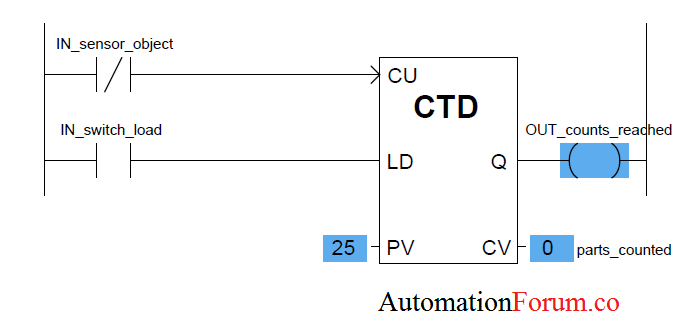# PLC Counter instructions | Example for counter logicsA counter is a PLC instruction that either increment (counts up) or decrements (counts down) an integer number value when prompted by the transition of a bit from 0 to 1 (“false” to “true”). Counter instructions come in three basic types: up counters, down counters, and up/down counters.

For both UP and Down counters there is only one trigger is needed to start the counting, but for up/down counter it is needed two triggers, one for up and other for down counter.

# UP counters:

Up counter is an increment counter. which means it counts “up” with each off-to-on transition input to its “CU” input.Whenever there is a true value entry in the CU input, the counter will turn on and CV will display the count. This counter output must be activated whenever the current value is equal to or greater than the preset value (Q is active if CV ≥ PV). The counter output instruction will increase by 1 each time the counted event occurs.

### Example program for UP counter:• Operating pushbutton PB1 provides the off-to-on transition pulses that are counted by the counter.
• The preset value of the counter is set to 7.
• Each false-to-true transition of rung 1 increases the counter’s accumulated value by 1.
• After 7 pulses, or counts, when the preset counter value equals the accumulated counter value, output DN is energized.
•  As a result, rung 2 becomes true and energizes output O:2/0 to switch the red pilot light on.
•  At the same time, rung 3 becomes false and de-energizes output O:2/1 to switch the green pilot light off.
• The counter is reset by closing pushbutton PB2, which makes rung 4 true and resets the accumulated count to zero.
• Counting can resume when rung 4 goes false again.

# Down counter:The down-counter instruction will count down or decrease by 1 each time the counted event occurs. Each time the countdown event occurs, the accumulated value decreases. Normally, the down counter is used together with the ascending counter to form an up / down counter.

### Example for Down counter:

One application for an up/down-counter is to keep count of the cars that enter and leave a parking garage. A typical PLC program that could be used to implement this. The operation of the program can be summarized as follows:• As a car enters, the enter switch triggers the up counter output instruction and increments the accumulated count by 1.
•   As a car leaves, the exit switch triggers the down counter output instruction and decrements the accumulated count by 1.
•  Because both the up- and down-counters have the same address, C5:1, the accumulated value will be the same in both instructions as well as the preset.
• Whenever the accumulated value of 150 equals the preset value of 150, the counter output is energized by the done bit to light up the Lot Full sign.
• A reset button has been provided to reset the accumulated count.

# Up-Down counter:

Up-Down counter do both the function of Up and Down counters:The counter will increase (count up) when sensor B de-energizes only if sensor A is already in the deactivated state (ie beam A breaks before B). The counter will decrease (countdown) when sensor A is de-energized only if sensor B is already in the de-energized state (ie, beam B breaks before A).

The up / down counter has a “reset” input (R) and a “load” (“LD”) input to force the current value. Activating the reset pin forces the counter to set zero, activating the load input forces the current value of the counter to the preset value (PV).

### Example program for Up-Down counter:• Separate count-up and count-down inputs are provided.
• Assuming the preset value of the counter is 3 and the accumulated count is 0, pulsing the count-up input (PB1) three times will switch the output light from off to on.
• This particular PLC counter keeps track of the number of counts received above the preset value. As a result, three additional pulses of the count-up input (PB1) produce an accumulated value of 6 but no change in the output.
• If the count-down input (PB2) is now pulsed four times, the accumulated count is reduced to 2 (6 2 4). As a result, the accumulated count drops below the preset count and the output light switches from on to off.
• Pulsing the reset input (PB3) at any time will reset the accumulated count to 0 and turn the output light off.